Courses

# Test: Steam Turbines -2

## 10 Questions MCQ Test Topicwise Question Bank for Mechanical Engineering | Test: Steam Turbines -2

Description
This mock test of Test: Steam Turbines -2 for Mechanical Engineering helps you for every Mechanical Engineering entrance exam. This contains 10 Multiple Choice Questions for Mechanical Engineering Test: Steam Turbines -2 (mcq) to study with solutions a complete question bank. The solved questions answers in this Test: Steam Turbines -2 quiz give you a good mix of easy questions and tough questions. Mechanical Engineering students definitely take this Test: Steam Turbines -2 exercise for a better result in the exam. You can find other Test: Steam Turbines -2 extra questions, long questions & short questions for Mechanical Engineering on EduRev as well by searching above.
QUESTION: 1

Solution:
QUESTION: 2

Solution:
QUESTION: 3

### Blading efficiency is defined as the ratio of

Solution:
QUESTION: 4

The optimum value of nozzle angle lies within the range

Solution:

The lower is the nozzle angle, higher is the blading efficiency. However, too low a nozzle angle may cause energy loss at blade inlet. Therefore, the nozzle angle low has to be maintained within a certain range, which varies from 16° to 22°

QUESTION: 5

If nozzle angle is 30°, the De Laval turbine will have a maximum efficiency of

Solution: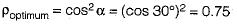QUESTION: 6

Steam enters a De LavaI steam turbine with an outlet velocity of 25 m/s and leaves with an outlet velocity of 5 m/s. The work done by 1 kg of steam is

Solution: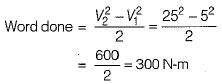QUESTION: 7

A single stage impulse turbine with a diameter of 100 cm runs at 3000 rpm. if the blade speed ratio is 0.45, the inlet velocity will be

Solution: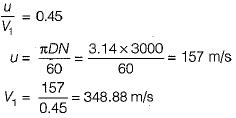QUESTION: 8

For a single stage impulse turbine with nozzle angle 15°, rotor diameter 2 m and speed 3000 rpm, the optimum velocity of steam in m/s is:
[Take cos15° = 0.966, sin15° = 0.258]

Solution: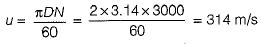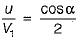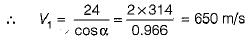QUESTION: 9

For an impulse turbine, the nozzle efficiency is 90%, the blade velocity ratio is 0.5 and the mean blade velocity is 150 m/s. This corresponds to heat drop in the nozzle equal to

Solution: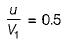∴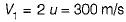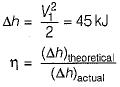∴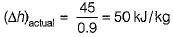QUESTION: 10

De Laval turbine is

Solution: